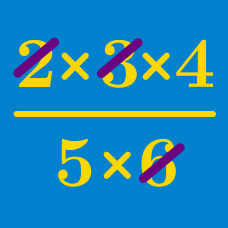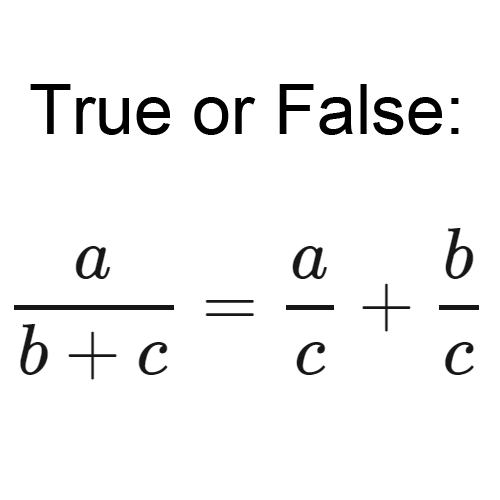Algebra

# Properties of Arithmetic

## Intro

Applying the distributive property, combining like terms, and/or cancelling common factors can turn a mathematical monster into a friend.

Expect to see and learn how to solve questions like this one:The properties of arithmetic govern how to approach numbers and operations in all sorts of contexts. These operations can be described based on how they behave. Can the order of the operands be switched? Does it matter which order you choose if you apply the operation twice? How do these operations interact with and have priority over one another?

These questions are addressed by identifying properties common to many functions. Understanding how these properties describe basic arithmetic operations can help lead to a deeper understand of how the many and varied functions of algebra behave.

×